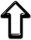﻿ Antonio Alarcon

# Research (Updated at November 2019)

My research is included in the field of Geometric Analysis, being Minimal Surface Theory my main area of expertise. I have focused on the study of minimal surfaces in $$\mathbb{R}^n$$ $$(n\geq 3)$$ by using both classical and modern Complex Analytic tools; in particular, the theory of Oka manifolds.
I have also studied other related topics, such as harmonic mappings between Riemannian manifolds, constant curvature surfaces in the Euclidean space $$\mathbb{R}^3$$, constant mean curvature $$1$$ surfaces in the Hyperbolic space $$\mathbb{H}^3$$, and, mainly, holomorphic curves in complex spaces. I have focused on the study of holomorphic null curves in complex Euclidean spaces and, more recently, holomorphic Legendrian curves in complex spaces and complex hypersurfaces in the complex Euclidean space $$\mathbb{C}^n$$ $$(n\geq 2)$$.

In 2016 my profile was included in the index of Emerging Stars of the University of Granada.

## Organization of Events